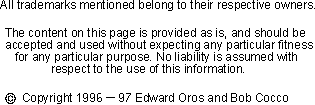The cubical quad antenna is a four sided antenna. In the standard quad design, each side of the quad is about a quarter wave length long. The actual antenna lengths depend on various factors. Some of these factors are:the height above ground, the diameter of the wire, nearby structures, the effects of other antennas in the area and even the conductivity of the soil.

This page allows you to calculate the length for a single element wire quad. It uses the standard formula, 306.324 / f MHz to calculate the quad's length. If you've experimented with quads before and know of a better formula for your QTH, feel free to change the formula to suit.
Enter the formula for the antenna calculation

Divided by Freq MHz